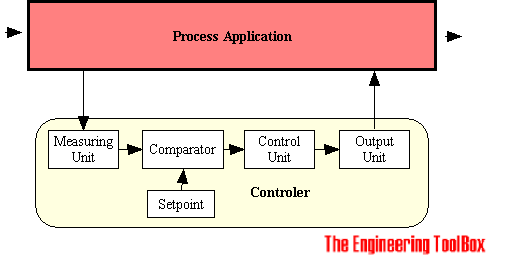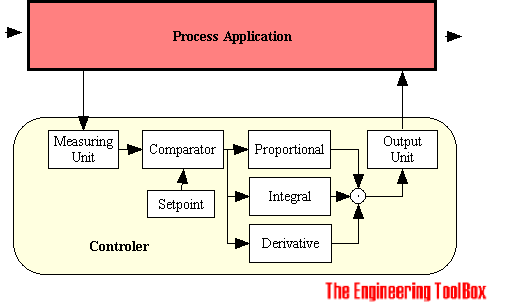Engineering ToolBox - Resources, Tools and Basic Information for Engineering and Design of Technical Applications!

# Process Controllers - P, PI & PID

## Proportional, integrating and derivative process controllers.

### Basic Controller

The Basic Controller for an application can be visualized asThe controller consists of

• a measuring unit with an appropriate instrument to measure the state of process, a temperature transmitter, pressure transmitter or similar
• a input set point device to set the desired value
• a comparator for comparing the measured value with the set point, calculating the difference or error between the two
• a control unit to calculate the output magnitude and direction to compensate the deviation from the desired value
• a output unit converting the output from the controller to physical action, a control valve, a motor or similar

### Controller Principles

The Control Units are in general build on the control principles

• proportional controller
• integral controller
• derivative controller### Proportional Controller (P-Controller)

One of the most used controllers is the Proportional Controller (P-Controller) who produce an output action that is proportional to the deviation between the set point and the measured process value.

OP = -kP Er                           (1)

where

OP = output proportional controller

kP = proportional gain or action factor of the controller

Er = error or deviation between the set point value and the measured value

The gain or action factor - kP

• influence on the output with a magnitude of kP
• determines how fast the system responds. If the value is too large the system will be in danger to oscillate and/or become unstable. If the value is too small the system error or deviation from set point will be very large
• can be regarded linear only for very small variations

The gain kP can be expressed as

kP = 100 / P                        (1b)

where

P = proportional band

The proportional band P, express the value necessary for 100% controller output. If P = 0, the gain or action factor kP would be infinity - the control action would be ON/OFF.

Note! A proportional controller will have the effect of reducing the rise time and will reduce, but never eliminate, the steady-state error.

### Integral Controller (I-Controller)

With integral action, the controller output is proportional to the amount of time the error is present. Integral action eliminates offset.

OI = - kI Σ(Er dt)                        (2)

where

OI = output integrating controller

kI = integrating gain or action factor of the controller

dt = time sample

The integral controller produce an output proportional to the summarized deviation between the set point and measured value and integrating gain or action factor.

Integral controllers tend to respond slowly at first, but over a long period of time they tend to eliminate errors.

The integral controller eliminates the steady-state error, but may make the transient response worse. The controller may be unstable.

The integral regulator may also cause problems during shutdowns and start up as a result of the integral saturation or wind up effect. An integrating regulator with over time deviation (typical during plant shut downs) will summarize the output to +/- 100%. During start up the output is set to 100%m which may be catastrophic.

### Derivative Controller (D-Controller)

With derivative action, the controller output is proportional to the rate of change of the measurement or error. The controller output is calculated by the rate of change of the deviation or error with time.

OD = - kD dEr / dt                           (3)

where

OD = output derivative controller

kD = derivative gain or action factor of the controller

dEr = deviation change over time sample dt

dt = time sample

The derivative or differential controller is never used alone. With sudden changes in the system the derivative controller will compensate the output fast. The long term effects the controller allow huge steady state errors.

A derivative controller will in general have the effect of increasing the stability of the system, reducing the overshoot, and improving the transient response.

### Proportional, Integral, Derivative Controller (PID-Controller)

The functions of the individual proportional, integral and derivative controllers complements each other. If they are combined its possible to make a system that responds quickly to changes (derivative), tracks required positions (proportional), and reduces steady state errors (integral).

Note that these correlations may not be exactly accurate, because P, I and D are dependent of each other. Changing one of these variables can change the effect of the other two.

PID - Proportional, Integral, Derivative Controller
Controller ResponseRise TimeOvershootSettling TimeSteady State Error
P Decrease Increase Small Change Decrease
I Decrease Increase Increase Eliminate
D Small Change Decrease Decrease Small Change

## Related Topics

• ### Control Valves

Control Valve terminology, bodies, trim, flow characteristics, Cv and Kv sizing, noise, actuators and positioners
• ### Process Control

Instrumentation and process control systems - design and documentation.

## Related Documents

• ### Control Valves - Flow Characteristics

Flow capacities vs. stem opening.
• ### Control Valves - Sizing and Selection

Sizing control valves to match process requirements.
• ### Gases - Control Valve Calculator

An online control valve - Cv - calculator for gases.
• ### ISA Instrumentation Codes used in Process Control Systems

The ISA standards and symbols are important for the P&IDs and documents describing the process control system.
• ### P&ID - Piping and Instrumentation Diagram

Schematic illustration of a functional relationship between piping, instrumentation and system components.
• ### P&ID Diagram - Online Drawing Tool

Draw P&ID diagrams online in the browser with Google Docs.
• ### PFD - Process Flow Diagram

The Process Flow Diagram (PFD) is a schematic illustration of a system.
• ### PFD - Process Flow Diagram - Online Drawing Tool

Draw Process Flow Diagrams with this online Google Docs drawing application.
• ### Process Control - Common Terms

Common terms used in the process control terminology.
• ### Process Instrumentation - ISA Codes

ISA process instrumentation codes - and combinations.
• ### Signal Transmission - Standards

Standard signals used for transmission from sensor to controller.
• ### Water Control Valves - Kv Calculation

Designing water process control valves and their Kv values.

## Engineering ToolBox - SketchUp Extension - Online 3D modeling!

Add standard and customized parametric components - like flange beams, lumbers, piping, stairs and more - to your Sketchup model with the Engineering ToolBox - SketchUp Extension - enabled for use with older versions of the amazing SketchUp Make and the newer "up to date" SketchUp Pro . Add the Engineering ToolBox extension to your SketchUp Make/Pro from the Extension Warehouse !

We don't collect information from our users. More about

## Citation

• The Engineering ToolBox (2003). Process Controllers - P, PI & PID. [online] Available at: https://www.engineeringtoolbox.com/process-controllers-d_499.html [Accessed Day Month Year].

Modify the access date according your visit.

10.2.10